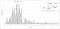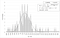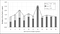# Understanding the nature of Venture Capital returns

To understand the driving forces behind Venture Capital returns I analyze the returns obtained by the California Public Employees Retirement System (CalPERS) out of 135 investments as a Limited Partner (LP) in VC Funds from 1999 to 2009. When plotting the data in a frequency distribution and fitting a Log-normal function to the experimental data, two intrinsic factors that seem to play a big role in VC become apparent: Randomness and Geometric Growth.

The analysis shows the following results:

• Mean TVPI (Total Value to Paid In) = 1,57
• Probability of TVPI > 1 = 75%
• Mean net IRR* (Internal Rate of Return) = 8,29%
• Average time that the investor’s capital is held within the Fund taking into account staged capital calls and distributions = 5,7 years
• The Time to Liquidity is longer than expected: Some VC Funds take as long as 16 years until the Fund is fully liquidated, i.e. when the ratios TVPI and DPI (Distributed to Paid In) converge.
• Both the TVPI and IRR distributions can be fairly well represented using Log-normal distributions in the range 0,6 ≤ TVPI ≤ 4 and -14% ≤ IRR ≤ 25%, respectively.
• In my opinion, the fact that a Log-normal distribution fits well to the experimental data implies that two factors that are intrinsic to this type of distributions might play a big role in VC: Randomness and Geometric Growth.

# Frequency distribution of the TVPIFig.1 Frequency distribution of the TVPIs obtained by CalPERS in its investments in VC funds. The solid line is a Log-normal distribution fitted to the experimental data. Mean TVPI = 1,57, which is similar to the theoretical value of the fitted curve.

In Fig. 1 the frequency distribution of the returns obtained by CalPERS in its investments in VC Funds is shown. The TVPI is calculated as the net multiple of the invested capital by the LP after fees. This value is the sum of the distributions to the LPs and the residual value of the not yet liquidated assets held in the Fund. Despite using the TVPI as a proxy for VC returns in this post, note that this is not entirely true until the Funds have been fully liquidated and the TVPI and DPI converge (see Value Gap below).

The fact that the experimental data can be explained using a Log-normal distribution has strong implications in understanding the underlying dynamics that drive VC returns. A Log-normal distribution is usually the result of two factors playing together: Randomness and Geometric Growth.

• Geometric growth: The value growth in VC backed companies is geometric rather than arithmetic. This means that the impact of VC financing can be better expressed as a factor that multiplies the existing value previous to the round instead of adding to it.
• Randomness: The outcome of the decisions made both at the company level and at the Fund level are strongly influenced by chance.

Some additional conclusions of the analysis:

• The mean value is more relevant than the median (as expected in skewed distributions where outliers become very important).
• Although the Log-normal distribution is a good fit to the experimental data for TVPI ≤ 4, the theoretical curve fails to predict the larger amount of outliers shown in the data when TVPI > 4. In such range, a power law such as described in the latest analysis of the EIF could be a better fit.

# Frequency distribution of the IRRFig. 2Frequency distribution of IRR obtained by CalPERS in its investments in VC funds. The solid line is a Log-normal distribution fitted to the experimental data. Mean IRR = 8,29%. *Note that the Mean IRR cannot be calculated as standard arithmetic mean of the individual IRR of each Fund.

In Fig. 2, the distribution of the individual IRRs obtained by CalPERS in its investments in VC Funds is shown. Note that the IRR is calculated using the TVPI instead of DPI, which assumes that the VC managers report a fair estimate of the assets held in their Funds.

Some conclusions of the analysis:

• The average time that the investor’s capital is held within the Fund taking into account staged capital calls and distributions is<t> = 5,7 years
• *Using <t> = 5,7 years and Mean TVPI = 1,57 we can calculate that Mean IRR = 8,29%
• Note that the IRR curve is remarkably similar to a Normal distribution. In fact, it can be shown that for |IRR| ≤ 10%, the curve can be approximated as a normal distribution. Understanding the implications of that might be subject of another post ;-)

# Time Evolution of TVPI, DPI and Value Gap (TVPI-DPI)Fig. 3 Average values of TVPI (solid line), DPI (dark grey) and Value Gap [TVPI-DPI] (light grey) as a function of time since inception.

Fig. 3 shows the average values of TVPI, DPI and Value Gap (TVPI-DPI) for each of the vintage years (expressed as Time since inception). This graph is a proxy of how the metrics of the VC Funds evolve over time. Note, however, that this is not the time evolution of a single fund but the snapshot of 135 funds classified as per Time since inception and depicted simulating such a time evolution. The differences in performance among different vintage years make the results of this type of analysis less accurate.

Some conclusions of the analysis:

• It takes time to sell the assets of a VC Fund: The Gap between TVPI and DPI can take as long as 16 years to close
• The growth in TVPI after the 6th year since inception is limited while the DPI almost doubles
• Most of the DPI value is generated between the 6th and the 16th year since inception (no surprises here)

# Definitions and Footnotes

TVPI: The ratio of the current value of remaining investments within a fund (net of fees), plus the total value of all distributions to date, relative to the total amount of capital paid into the fund to date.

DPI: The ratio of money distributed to Limited Partners by the Fund, relative to contributions.

IRR: The discount rate at which the present value of future cash flows of an investment equals to the cost of the investment.

— — — — — — — — — — — — — — — — — — — — — — — — — — — — — — —

About myself: I’m VC @ Nauta Capital. I’d love to blog more often but I only do it when I’m not doing DD or helping our portfolio ;-)

Written by

Written by

## Guillem

#### Partner @ Nauta## More From Medium

Welcome to a place where words matter. On Medium, smart voices and original ideas take center stage - with no ads in sight. Watch
Follow all the topics you care about, and we’ll deliver the best stories for you to your homepage and inbox. Explore
Get unlimited access to the best stories on Medium — and support writers while you’re at it. Just \$5/month. Upgrade